## Determining the Amplitude and Period of a Sinusoidal Function

Any motion that repeats itself in a fixed time period is considered periodic motion and can be modeled by a sinusoidal function. The amplitude of a sinusoidal function is the distance from the midline to the maximum value, or from the midline to the minimum value. The midline is the average value. Sinusoidal functions oscillate above and below the midline, are periodic, and repeat values in set cycles. Recall from Graphs of the Sine and Cosine Functions that the period of the sine function and the cosine function is $\text{ }2\pi .\text{ }$ In other words, for any value of $\text{ }x$,

$\sin \left(x\pm 2\pi k\right)=\sin x\text{ and }\cos \left(x\pm 2\pi k\right)=\cos x\text{ where }k\text{ is an integer}$

### A General Note: Standard Form of Sinusoidal Equations

The general forms of a sinusoidal equation are given as

$y=A\sin \left(Bt-C\right)+D\text{ or }y=A\cos \left(Bt-C\right)+D$

where $\text{amplitude}=|A|,B$ is related to period such that the $\text{ period}=\frac{2\pi }{B},C\text{ }$ is the phase shift such that $\text{ }\frac{C}{B}\text{ }$ denotes the horizontal shift, and $\text{ }D\text{ }$ represents the vertical shift from the graph’s parent graph.

Note that the models are sometimes written as $\text{ }y=a\sin \left(\omega t\pm C\right)+D\text{ }$ or $\text{ }y=a\cos \left(\omega t\pm C\right)+D$, and period is given as $\text{ }\frac{2\pi }{\omega }$.

The difference between the sine and the cosine graphs is that the sine graph begins with the average value of the function and the cosine graph begins with the maximum or minimum value of the function.

### Example 1: Showing How the Properties of a Trigonometric Function Can Transform a Graph

Show the transformation of the graph of $\text{ }y=\sin x\text{ }$ into the graph of $\text{ }y=2\sin \left(4x-\frac{\pi }{2}\right)+2$.

### Solution

Consider the series of graphs in Figure 2 and the way each change to the equation changes the image.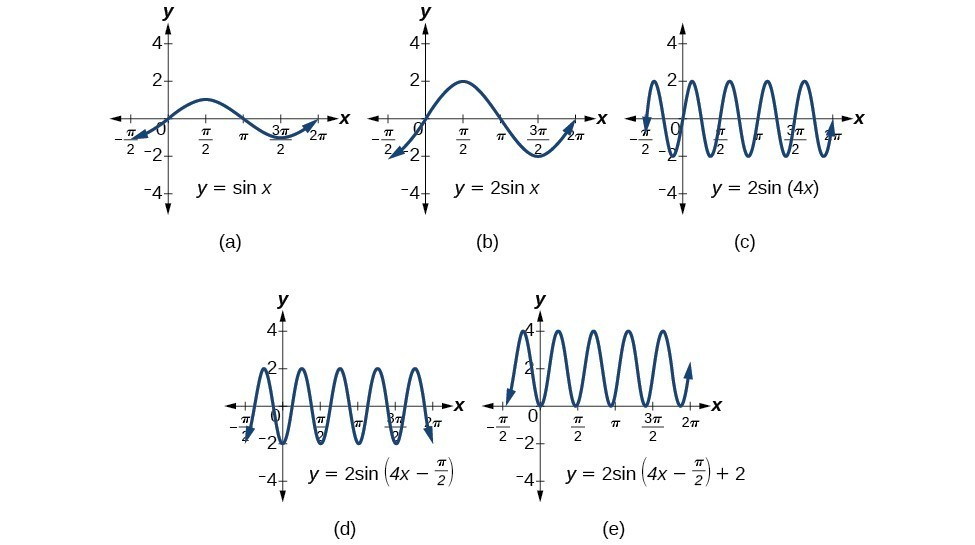Figure 2. (a) The basic graph of $\text{ }y=\sin x\text{ }$ (b) Changing the amplitude from 1 to 2 generates the graph of $\text{ }y=2\sin x.\text{ }$ (c) The period of the sine function changes with the value of $\text{ }B$, such that $\text{ period}=\frac{2\pi }{B}$. Here we have $\text{ }B=4$, which translates to a period of $\text{ }\frac{\pi }{2}$. The graph completes one full cycle in $\text{ }\frac{\pi }{2}\text{ }$ units. (d) The graph displays a horizontal shift equal to $\text{ }\frac{C}{B}$, or $\text{ }\frac{\frac{\pi }{2}}{4}=\frac{\pi }{8}$. (e) Finally, the graph is shifted vertically by the value of $\text{ }D$. In this case, the graph is shifted up by 2 units.

### Example 2: Finding the Amplitude and Period of a Function

Find the amplitude and period of the following functions and graph one cycle.

1. $y=2\sin \left(\frac{1}{4}x\right)$
2. $y=-3\sin \left(2x+\frac{\pi }{2}\right)$
3. $y=\cos x+3$

### Solution

We will solve these problems according to the models.

1. $y=2\sin \left(\frac{1}{4}x\right)\text{ }$ involves sine, so we use the form
$y=A\sin \left(Bt+C\right)+D$

We know that $\text{ }|A|\text{ }$ is the amplitude, so the amplitude is 2. Period is $\text{ }\frac{2\pi }{B}$, so the period is

$\begin{array}{l}\frac{2\pi }{B}=\frac{2\pi }{\frac{1}{4}}\hfill \\ \text{ }=8\pi \hfill \end{array}$

See the graph in Figure 3.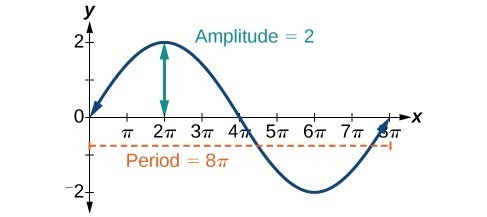Figure 3

2. $y=-3\sin \left(2x+\frac{\pi }{2}\right)\text{ }$ involves sine, so we use the form
$y=A\sin \left(Bt-C\right)+D$

Amplitude is $\text{ }|A|$, so the amplitude is $\text{ }|-3|=3$. Since $\text{ }A\text{ }$ is negative, the graph is reflected over the x-axis. Period is $\text{ }\frac{2\pi }{B}$, so the period is

$\frac{2\pi }{B}=\frac{2\pi }{2}=\pi$

The graph is shifted to the left by $\text{ }\frac{C}{B}=\frac{\frac{\pi }{2}}{2}=\frac{\pi }{4}\text{ }$ units.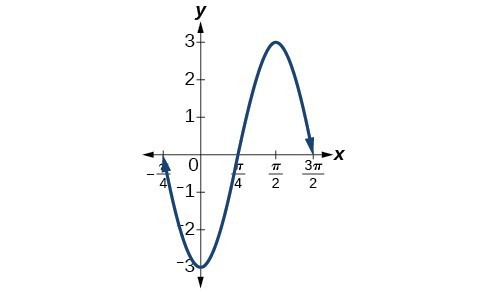Figure 4

3. $y=\cos x+3\text{ }$ involves cosine, so we use the form
$y=A\cos \left(Bt\pm C\right)+D$

Amplitude is $\text{ }|A|,\text{ }$ so the amplitude is 1. The period is $\text{ }2\pi .\text{ }$ This is the standard cosine function shifted up three units.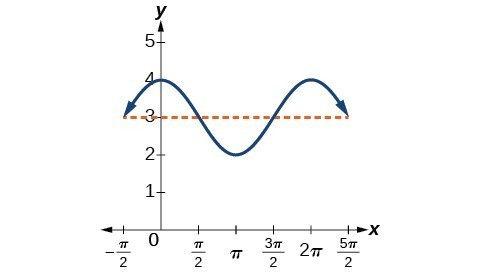Figure 5

### Try It 1

What are the amplitude and period of the function $\text{ }y=3\cos \left(3\pi x\right)?$

## Finding Equations and Graphing Sinusoidal Functions

One method of graphing sinusoidal functions is to find five key points. These points will correspond to intervals of equal length representing $\text{ }\frac{1}{4}\text{ }$ of the period. The key points will indicate the location of maximum and minimum values. If there is no vertical shift, they will also indicate x-intercepts. For example, suppose we want to graph the function $\text{ }y=\cos \theta$. We know that the period is $2\pi$, so we find the interval between key points as follows.

$\frac{2\pi }{4}=\frac{\pi }{2}$

Starting with $\text{ }\theta =0$, we calculate the first y-value, add the length of the interval $\text{ }\frac{\pi }{2}\text{ }$ to 0, and calculate the second y-value. We then add $\text{ }\frac{\pi }{2}\text{ }$ repeatedly until the five key points are determined. The last value should equal the first value, as the calculations cover one full period. Making a table similar to the one below, we can see these key points clearly on the graph shown in Figure 6.

 $\theta$ $0$ $\frac{\pi }{2}$ $\pi$ $\frac{3\pi }{2}$ $2\pi$ $y=\cos \theta$ $1$ $0$ $-1$ $0$ $1$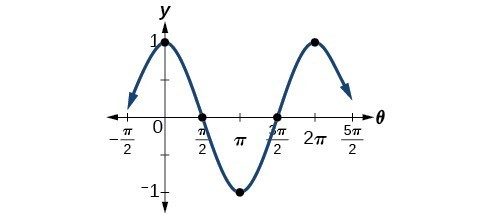Figure 6

### Example 3: Graphing Sinusoidal Functions Using Key Points

Graph the function $\text{ }y=-4\cos \left(\pi x\right)\text{ }$ using amplitude, period, and key points.

### Solution

The amplitude is $|-4|=4.$ The period is $\text{ }\frac{2\pi }{\omega }=\frac{2\pi }{\pi }=2.\text{ }$ (Recall that we sometimes refer to $\text{ }B\text{ }$ as $\omega$.) One cycle of the graph can be drawn over the interval $\text{ }\left[0,2\right].\text{ }$ To find the key points, we divide the period by 4. Make a table similar to the one below, starting with $\text{ }x=0\text{ }$ and then adding $\text{ }\frac{1}{2}\text{ }$ successively to $\text{ }x\text{ }$ and calculate $\text{ }y.\text{ }$ See the graph in Figure 7.

 $x$ $0$ $\frac{1}{2}$ $1$ $\frac{3}{2}$ $2$ $y=-4\cos \left(\pi x\right)$ $-4$ $0$ $4$ $0$ $-4$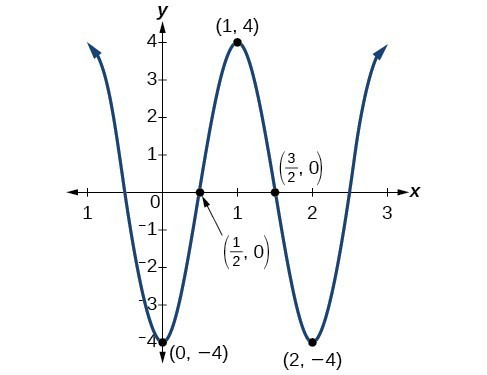Figure 7

### Try It 2

Graph the function $\text{ }y=3\sin \left(3x\right)\text{ }$ using the amplitude, period, and five key points.

Solution

## Modeling Periodic Behavior

We will now apply these ideas to problems involving periodic behavior.

### Example 4: Modeling an Equation and Sketching a Sinusoidal Graph to Fit Criteria

The average monthly temperatures for a small town in Oregon are given in the table below. Find a sinusoidal function of the form $y=A\sin \left(Bt-C\right)+D$ that fits the data (round to the nearest tenth) and sketch the graph.

Month Temperature, ${}^{\text{o}}\text{F}$
January 42.5
February 44.5
March 48.5
April 52.5
May 58
June 63
July 68.5
August 69
September 64.5
October 55.5
November 46.5
December 43.5

### Solution

Recall that amplitude is found using the formula

$A=\frac{\text{largest value }-\text{smallest value}}{2}$

Thus, the amplitude is

$\begin{array}{l}\hfill \\ |A|=\frac{69 - 42.5}{2}\hfill \\ \text{ }=13.25\hfill \end{array}$

The data covers a period of 12 months, so $\frac{2\pi }{B}=12$ which gives $B=\frac{2\pi }{12}=\frac{\pi }{6}$.

The vertical shift is found using the following equation.

$D=\frac{\text{highest value}+\text{lowest value}}{2}$

Thus, the vertical shift is

$\begin{array}{l}\\ \begin{array}{l}D=\frac{69+42.5}{2}\hfill \\ \text{ }=55.8\hfill \end{array}\end{array}$

So far, we have the equation $y=13.3\sin \left(\frac{\pi }{6}x-C\right)+55.8$.

To find the horizontal shift, we input the $x$ and $y$ values for the first month and solve for $C$.

$\begin{array}{ll}\text{ }42.5=13.3\sin \left(\frac{\pi }{6}\left(1\right)-C\right)+55.8\hfill & \hfill \\ -13.3=13.3\sin \left(\frac{\pi }{6}-C\right)\hfill & \hfill \\ \text{ }-1=\sin \left(\frac{\pi }{6}-C\right)\hfill & \sin \theta =-1\to \theta =-\frac{\pi }{2}\hfill \\ \frac{\pi }{6}-C=-\frac{\pi }{2}\hfill & \hfill \\ \frac{\pi }{6}+\frac{\pi }{2}=C\hfill & \hfill \\ \text{ }=\frac{2\pi }{3}\hfill & \hfill \end{array}$

We have the equation $y=13.3\sin \left(\frac{\pi }{6}x-\frac{2\pi }{3}\right)+55.8$. See the graph in Figure 8.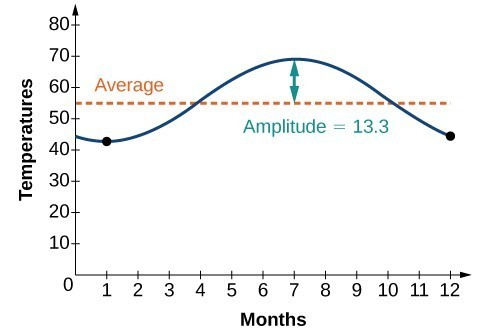Figure 8

### Example 5: Describing Periodic Motion

The hour hand of the large clock on the wall in Union Station measures 24 inches in length. At noon, the tip of the hour hand is 30 inches from the ceiling. At 3 PM, the tip is 54 inches from the ceiling, and at 6 PM, 78 inches. At 9 PM, it is again 54 inches from the ceiling, and at midnight, the tip of the hour hand returns to its original position 30 inches from the ceiling. Let $y$ equal the distance from the tip of the hour hand to the ceiling $x$ hours after noon. Find the equation that models the motion of the clock and sketch the graph.

### Solution

Begin by making a table of values as shown in the table below.

$x$ $y$ Points to plot
Noon 30 in $\left(0,30\right)$
3 PM 54 in $\left(3,54\right)$
6 PM 78 in $\left(6,78\right)$
9 PM 54 in $\left(9,54\right)$
Midnight 30 in $\left(12,30\right)$

To model an equation, we first need to find the amplitude.

$\begin{array}{l}\hfill \\ \begin{array}{l}|A|=|\frac{78 - 30}{2}|\hfill \\ \text{ }=24\hfill \end{array}\hfill \end{array}$

The clock’s cycle repeats every 12 hours. Thus,

$\begin{array}{l}\\ \begin{array}{l}B=\frac{2\pi }{12}\hfill \\ \text{ }=\frac{\pi }{6}\hfill \end{array}\end{array}$

The vertical shift is

$\begin{array}{l}\\ \begin{array}{l}D=\frac{78+30}{2}\hfill \\ \text{ }=54\hfill \end{array}\end{array}$

There is no horizontal shift, so $C=0$. Since the function begins with the minimum value of $y$ when $x=0$ (as opposed to the maximum value), we will use the cosine function with the negative value for $A$. In the form $y=A\cos \left(Bx\pm C\right)+D$, the equation is

$y=-24\cos \left(\frac{\pi }{6}x\right)+54$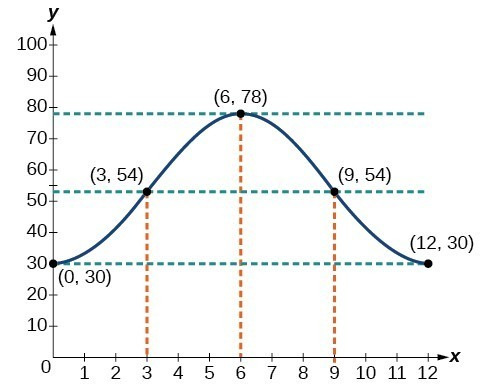Figure 9

### Example 6: Determining a Model for Tides

The height of the tide in a small beach town is measured along a seawall. Water levels oscillate between 7 feet at low tide and 15 feet at high tide. On a particular day, low tide occurred at 6 AM and high tide occurred at noon. Approximately every 12 hours, the cycle repeats. Find an equation to model the water levels.

### Solution

As the water level varies from 7 ft to 15 ft, we can calculate the amplitude as

$\begin{array}{l}\hfill \\ \begin{array}{l}|A|=|\frac{\left(15 - 7\right)}{2}|\hfill \\ \text{ }=4\hfill \end{array}\hfill \end{array}$

The cycle repeats every 12 hours; therefore, $B$ is

$\frac{2\pi }{12}=\frac{\pi }{6}$

There is a vertical translation of $\frac{\left(15+8\right)}{2}=11.5$. Since the value of the function is at a maximum at $t=0$, we will use the cosine function, with the positive value for $A$.

$y=4\cos \left(\frac{\pi }{6}\right)t+11$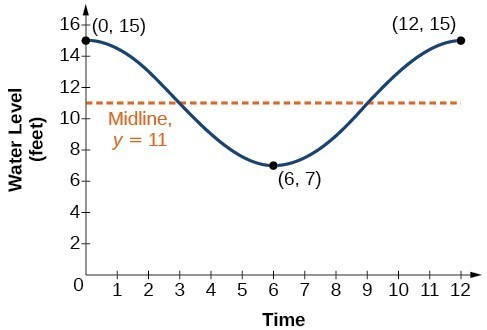Figure 10

### Try It 3

The daily temperature in the month of March in a certain city varies from a low of $24^\circ\text{F}$ to a high of $40^\circ\text{F}$. Find a sinusoidal function to model daily temperature and sketch the graph. Approximate the time when the temperature reaches the freezing point $32^\circ\text{F}$. Let $t=0$ correspond to noon.

Solution

### Example 7: Interpreting the Periodic Behavior Equation

The average person’s blood pressure is modeled by the function $f\left(t\right)=20\sin \left(160\pi t\right)+100$, where $f\left(t\right)$ represents the blood pressure at time $t$, measured in minutes. Interpret the function in terms of period and frequency. Sketch the graph and find the blood pressure reading.

### Solution

The period is given by

$\begin{array}{l}\\ \begin{array}{l}\frac{2\pi }{\omega }=\frac{2\pi }{160\pi }\hfill \\ \text{ }=\frac{1}{80}\hfill \end{array}\end{array}$

In a blood pressure function, frequency represents the number of heart beats per minute. Frequency is the reciprocal of period and is given by

$\begin{array}{c}\frac{\omega }{2\pi }=\frac{160\pi }{2\pi }\\ =80\end{array}$

See the graph in Figure 11.

The blood pressure reading on the graph is $\frac{120}{80}\text{ }\left(\frac{\text{maximum}}{\text{minimum}}\right)$.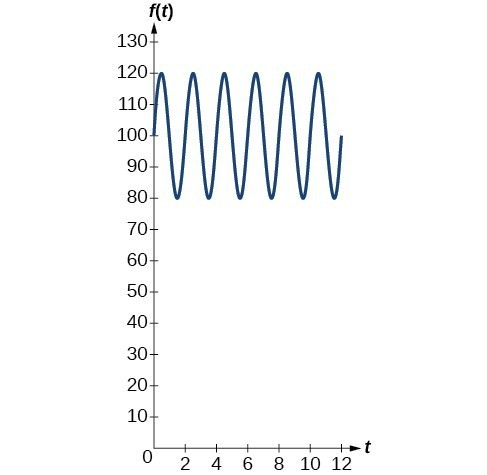Figure 11

### Analysis of the Solution

Blood pressure of $\frac{120}{80}$ is considered to be normal. The top number is the maximum or systolic reading, which measures the pressure in the arteries when the heart contracts. The bottom number is the minimum or diastolic reading, which measures the pressure in the arteries as the heart relaxes between beats, refilling with blood. Thus, normal blood pressure can be modeled by a periodic function with a maximum of 120 and a minimum of 80.

## Modeling Harmonic Motion Functions

Harmonic motion is a form of periodic motion, but there are factors to consider that differentiate the two types. While general periodic motion applications cycle through their periods with no outside interference, harmonic motion requires a restoring force. Examples of harmonic motion include springs, gravitational force, and magnetic force.

### Simple Harmonic Motion

A type of motion described as simple harmonic motion involves a restoring force but assumes that the motion will continue forever. Imagine a weighted object hanging on a spring, When that object is not disturbed, we say that the object is at rest, or in equilibrium. If the object is pulled down and then released, the force of the spring pulls the object back toward equilibrium and harmonic motion begins. The restoring force is directly proportional to the displacement of the object from its equilibrium point. When $t=0,d=0$.

### A General Note: Simple Harmonic Motion

We see that simple harmonic motion equations are given in terms of displacement:

$d=a\cos \left(\omega t\right)\text{ or }d=a\sin \left(\omega t\right)$

where $|a|$ is the amplitude, $\frac{2\pi }{\omega }$ is the period, and $\frac{\omega }{2\pi }$ is the frequency, or the number of cycles per unit of time.

### Example 8: Finding the Displacement, Period, and Frequency, and Graphing a Function

For the given functions,

1. Find the maximum displacement of an object.
2. Find the period or the time required for one vibration.
3. Find the frequency.
4. Sketch the graph.
1. $y=5\sin \left(3t\right)$
2. $y=6\cos \left(\pi t\right)$
3. $y=5\cos \left(\frac{\pi }{2}t\right)$

### Solution

1. $y=5\sin \left(3t\right)$
1. The maximum displacement is equal to the amplitude, $|a|$, which is 5.
2. The period is $\frac{2\pi }{\omega }=\frac{2\pi }{3}$.
3. The frequency is given as $\frac{\omega }{2\pi }=\frac{3}{2\pi }$.
4. See Figure 12. The graph indicates the five key points.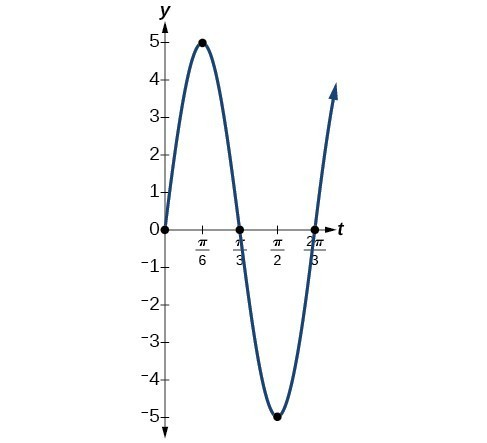Figure 12

2. $y=6\cos \left(\pi t\right)$
1. The maximum displacement is $6$.
2. The period is $\frac{2\pi }{\omega }=\frac{2\pi }{\pi }=2$.
3. The frequency is $\frac{\omega }{2\pi }=\frac{\pi }{2\pi }=\frac{1}{2}$.
4. See Figure 13.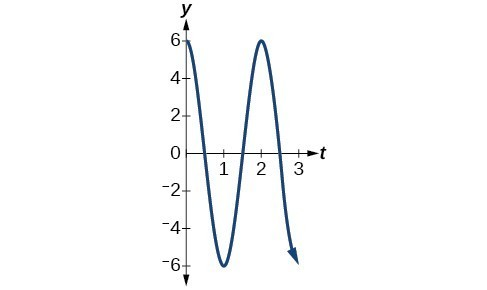Figure 13.

3. $y=5\cos \left(\frac{\pi }{2}\right)t$
1. The maximum displacement is $5$.
2. The period is $\frac{2\pi }{\omega }=\frac{2\pi }{\frac{\pi }{2}}=4$.
3. The frequency is $\frac{1}{4}$.
4. See Figure 14.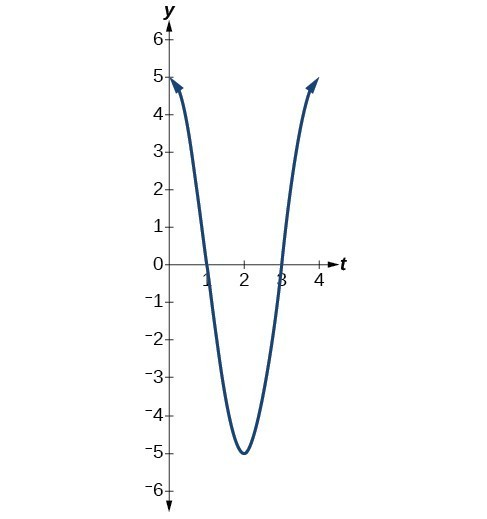Figure 14

## Damped Harmonic Motion

In reality, a pendulum does not swing back and forth forever, nor does an object on a spring bounce up and down forever. Eventually, the pendulum stops swinging and the object stops bouncing and both return to equilibrium. Periodic motion in which an energy-dissipating force, or damping factor, acts is known as damped harmonic motion. Friction is typically the damping factor.

In physics, various formulas are used to account for the damping factor on the moving object. Some of these are calculus-based formulas that involve derivatives. For our purposes, we will use formulas for basic damped harmonic motion models.

### A General Note: Damped Harmonic Motion

In damped harmonic motion, the displacement of an oscillating object from its rest position at time $t$ is given as

$f\left(t\right)=a{e}^{-ct}\sin \left(\omega t\right)\text{or} f\left(t\right)=a{e}^{-ct}\cos \left(\omega t\right)$

where $c$ is a damping factor, $|a|$ is the initial displacement and $\frac{2\pi }{\omega }$ is the period.

### Example 8: Modeling Damped Harmonic Motion

Model the equations that fit the two scenarios and use a graphing utility to graph the functions: Two mass-spring systems exhibit damped harmonic motion at a frequency of $0.5$ cycles per second. Both have an initial displacement of 10 cm. The first has a damping factor of $0.5$ and the second has a damping factor of $0.1$.

### Solution

At time $t=0$, the displacement is the maximum of 10 cm, which calls for the cosine function. The cosine function will apply to both models.

We are given the frequency $f=\frac{\omega }{2\pi }$ of 0.5 cycles per second. Thus,

$\begin{array}{l}\text{ }\frac{\omega }{2\pi }=0.5\hfill \\ \text{ }\omega =\left(0.5\right)2\pi \hfill \\ \text{ }=\pi \hfill \end{array}$

The first spring system has a damping factor of $c=0.5$. Following the general model for damped harmonic motion, we have

$f\left(t\right)=10{e}^{-0.5t}\cos \left(\pi t\right)$

Figure 15 models the motion of the first spring system.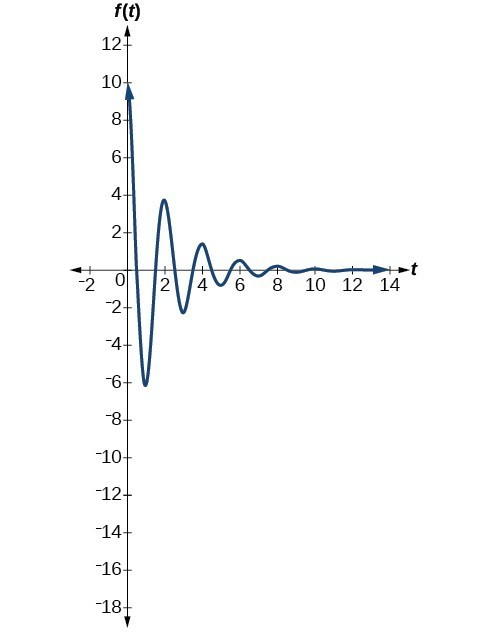Figure 15

The second spring system has a damping factor of $c=0.1$ and can be modeled as

$f\left(t\right)=10{e}^{-0.1t}\cos \left(\pi t\right)$

Figure 16 models the motion of the second spring system.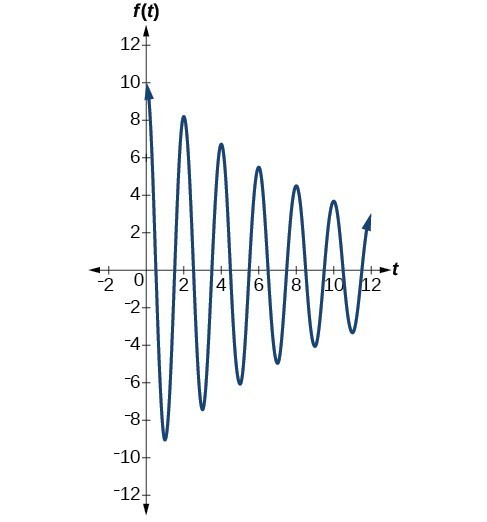Figure 16

### Analysis of the Solution

Notice the differing effects of the damping constant. The local maximum and minimum values of the function with the damping factor $c=0.5$ decreases much more rapidly than that of the function with $c=0.1$.

### Example 9: Finding a Cosine Function that Models Damped Harmonic Motion

Find and graph a function of the form $y=a{e}^{-ct}\cos \left(\omega t\right)$ that models the information given.

1. $a=20,c=0.05,p=4$
2. $a=2,c=1.5,f=3$

### Solution

Substitute the given values into the model. Recall that period is $\frac{2\pi }{\omega }$ and frequency is $\frac{\omega }{2\pi }$.

1. $y=20{e}^{-0.05t}\cos \left(\frac{\pi }{2}t\right)$. See Figure 17.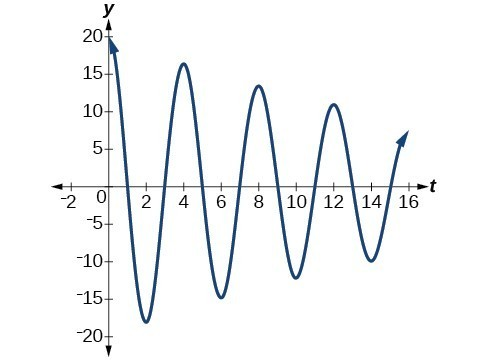Figure 17

2. $y=2{e}^{-1.5t}\cos \left(6\pi t\right)$. See Figure 18.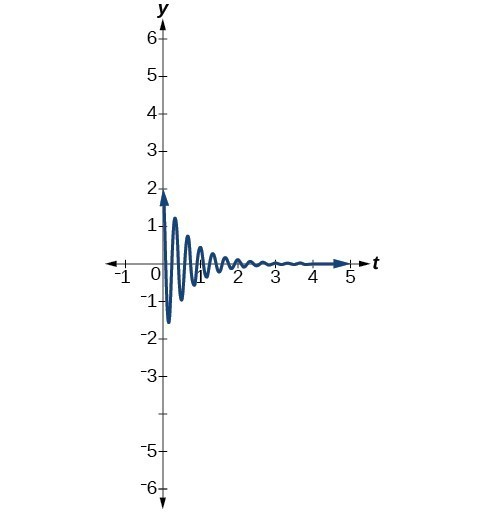Figure 18

### Try It 4

The following equation represents a damped harmonic motion model: $\text{ }f\left(t\right)=5{e}^{-6t}\cos \left(4t\right)$
Find the initial displacement, the damping constant, and the frequency.

Solution

### Example 10: Finding a Sine Function that Models Damped Harmonic Motion

Find and graph a function of the form $y=a{e}^{-ct}\sin \left(\omega t\right)$ that models the information given.

1. $a=7,c=10,p=\frac{\pi }{6}$
2. $a=0.3,c=0.2,f=20$

### Solution

Calculate the value of $\omega$ and substitute the known values into the model.

1. As period is $\frac{2\pi }{\omega }$, we have
$\begin{array}{l}\text{ }\frac{\pi }{6}=\frac{2\pi }{\omega }\hfill \\ \text{ }\omega \pi =6\left(2\pi \right)\hfill \\ \text{ }\omega =12\hfill \end{array}$

The damping factor is given as 10 and the amplitude is 7. Thus, the model is $y=7{e}^{-10t}\sin \left(12t\right)$. See Figure 19.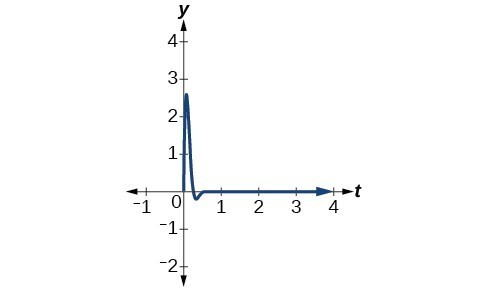Figure 19

2. As frequency is $\frac{\omega }{2\pi }$, we have
$\begin{array}{l}\text{ }20=\frac{\omega }{2\pi }\hfill \\ 40\pi =\omega \hfill \end{array}$

The damping factor is given as $0.2$ and the amplitude is $0.3$. The model is $y=0.3{e}^{-0.2t}\sin \left(40\pi t\right)$. See Figure 20.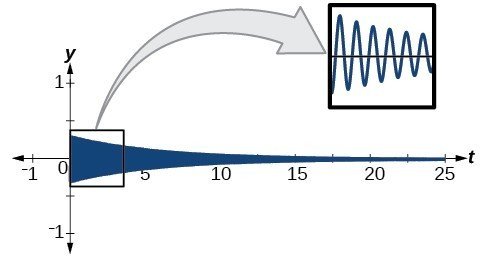Figure 20

### Analysis of the Solution

A comparison of the last two examples illustrates how we choose between the sine or cosine functions to model sinusoidal criteria. We see that the cosine function is at the maximum displacement when $t=0$, and the sine function is at the equilibrium point when $t=0$. For example, consider the equation $y=20{e}^{-0.05t}\cos \left(\frac{\pi }{2}t\right)$ from Example 9. We can see from the graph that when $t=0,y=20$, which is the initial amplitude. Check this by setting $t=0$ in the cosine equation:

$\begin{array}{l}\\ \begin{array}{l}y=20{e}^{-0.05\left(0\right)}\cos \left(\frac{\pi }{2}\right)\left(0\right)\hfill \\ =20\left(1\right)\left(1\right)\hfill \\ =20\hfill \end{array}\end{array}$

Using the sine function yields

$\begin{array}{l}y=20{e}^{-0.05\left(0\right)}\sin \left(\frac{\pi }{2}\right)\left(0\right)\hfill \\ =20\left(1\right)\left(0\right)\hfill \\ =0\hfill \end{array}$

Thus, cosine is the correct function.

### Try It 5

Write the equation for damped harmonic motion given $a=10,c=0.5$, and $p=2$.

Solution

### Example 11: Modeling the Oscillation of a Spring

A spring measuring 10 inches in natural length is compressed by 5 inches and released. It oscillates once every 3 seconds, and its amplitude decreases by 30% every second. Find an equation that models the position of the spring $t$ seconds after being released.

### Solution

The amplitude begins at 5 in. and deceases 30% each second. Because the spring is initially compressed, we will write A as a negative value. We can write the amplitude portion of the function as

$A\left(t\right)=5{\left(1 - 0.30\right)}^{t}$

We put ${\left(1 - 0.30\right)}^{t}$ in the form ${e}^{ct}$ as follows:

$\begin{array}{l}0.7={e}^{c}\hfill \\ \text{ }c=\mathrm{ln}.7\hfill \\ \text{ }c=-0.357\hfill \end{array}$

Now let’s address the period. The spring cycles through its positions every 3 seconds, this is the period, and we can use the formula to find omega.

$\begin{array}{l}\hfill \\ 3=\frac{2\pi }{\omega }\hfill \\ \omega =\frac{2\pi }{3}\hfill \end{array}$

The natural length of 10 inches is the midline. We will use the cosine function, since the spring starts out at its maximum displacement. This portion of the equation is represented as

$y=\cos \left(\frac{2\pi }{3}t\right)+10$

Finally, we put both functions together. Our the model for the position of the spring at $t$ seconds is given as

$y=-5{e}^{-0.357t}\cos \left(\frac{2\pi }{3}t\right)+10$

See the graph in Figure 21.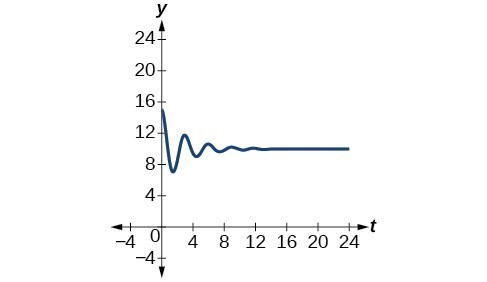Figure 21

### Try It 6

A mass suspended from a spring is raised a distance of 5 cm above its resting position. The mass is released at time $t=0$ and allowed to oscillate. After $\frac{1}{3}$ second, it is observed that the mass returns to its highest position. Find a function to model this motion relative to its initial resting position.

Solution

### Example 12: Finding the Value of the Damping Constant c According to the Given Criteria

A guitar string is plucked and vibrates in damped harmonic motion. The string is pulled and displaced 2 cm from its resting position. After 3 seconds, the displacement of the string measures 1 cm. Find the damping constant.

### Solution

The displacement factor represents the amplitude and is determined by the coefficient $a{e}^{-ct}$ in the model for damped harmonic motion. The damping constant is included in the term ${e}^{-ct}$. It is known that after 3 seconds, the local maximum measures one-half of its original value. Therefore, we have the equation

$a{e}^{-c\left(t+3\right)}=\frac{1}{2}a{e}^{-ct}$

Use algebra and the laws of exponents to solve for $c$.

$\begin{array}{ll} a{e}^{-c\left(t+3\right)}=\frac{1}{2}a{e}^{-ct}\hfill & \hfill \\ {e}^{-ct}\cdot {e}^{-3c}=\frac{1}{2}{e}^{-ct}\begin{array}{cccc}& & & \end{array}\hfill & \text{Divide out }a.\hfill \\ {e}^{-3c}=\frac{1}{2} \hfill & \text{Divide out }{e}^{-ct}.\hfill \\ {e}^{3c}=2\hfill & \text{Take reciprocals}.\hfill \end{array}$

Then use the laws of logarithms.

$\begin{array}{l}{e}^{3c}=2\hfill \\ \text{ }3c=\mathrm{ln}2\hfill \\ \text{ }c=\frac{\mathrm{ln}2}{3}\hfill \end{array}$

The damping constant is $\frac{\mathrm{ln}2}{3}$.

## Bounding Curves in Harmonic Motion

Harmonic motion graphs may be enclosed by bounding curves. When a function has a varying amplitude, such that the amplitude rises and falls multiple times within a period, we can determine the bounding curves from part of the function.

### Example 13: Graphing an Oscillating Cosine Curve

Graph the function $f\left(x\right)=\cos \left(2\pi x\right)\cos \left(16\pi x\right)$.

### Solution

The graph produced by this function will be shown in two parts. The first graph will be the exact function $f\left(x\right)$, and the second graph is the exact function $f\left(x\right)$ plus a bounding function. The graphs look quite different.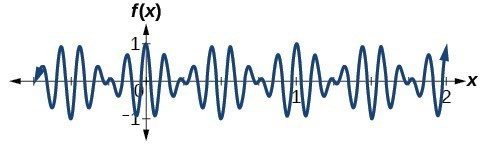Figure 22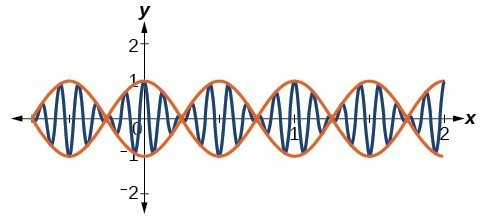Figure 23

### Analysis of the Solution

The curves $y=\cos \left(2\pi x\right)$ and $y=-\cos \left(2\pi x\right)$ are bounding curves: they bound the function from above and below, tracing out the high and low points. The harmonic motion graph sits inside the bounding curves. This is an example of a function whose amplitude not only decreases with time, but actually increases and decreases multiple times within a period.

## Key Equations

 Standard form of sinusoidal equation $y=A\sin \left(Bt-C\right)+D\text{or}y=A\cos \left(Bt-C\right)+D$ Simple harmonic motion $d=a\cos \left(\omega t\right)\text{ or }d=a\sin \left(\omega t\right)$ Damped harmonic motion $f\left(t\right)=a{e}^{-c}{}^{t}\sin \left(\omega t\right)\text{or}f\left(t\right)=a{e}^{-ct}\cos \left(\omega t\right)$

## Key Concepts

• Sinusoidal functions are represented by the sine and cosine graphs. In standard form, we can find the amplitude, period, and horizontal and vertical shifts.
• Use key points to graph a sinusoidal function. The five key points include the minimum and maximum values and the midline values.
• Periodic functions can model events that reoccur in set cycles, like the phases of the moon, the hands on a clock, and the seasons in a year.
• Harmonic motion functions are modeled from given data. Similar to periodic motion applications, harmonic motion requires a restoring force. Examples include gravitational force and spring motion activated by weight.
• Damped harmonic motion is a form of periodic behavior affected by a damping factor. Energy dissipating factors, like friction, cause the displacement of the object to shrink.
• Bounding curves delineate the graph of harmonic motion with variable maximum and minimum values.

## Glossary

damped harmonic motion
oscillating motion that resembles periodic motion and simple harmonic motion, except that the graph is affected by a damping factor, an energy dissipating influence on the motion, such as friction
simple harmonic motion
a repetitive motion that can be modeled by periodic sinusoidal oscillation

## Section Exercises

1. Explain what types of physical phenomena are best modeled by sinusoidal functions. What are the characteristics necessary?

2. What information is necessary to construct a trigonometric model of daily temperature? Give examples of two different sets of information that would enable modeling with an equation.

3. If we want to model cumulative rainfall over the course of a year, would a sinusoidal function be a good model? Why or why not?

4. Explain the effect of a damping factor on the graphs of harmonic motion functions.

For the following exercises, find a possible formula for the trigonometric function represented by the given table of values.

5.

 $x$ $y$ $0$ $-4$ $3$ $-1$ $6$ $2$ $9$ $-1$ $12$ $-4$ $15$ $-1$ $18$ $2$

6.

 $x$ $y$ $0$ $5$ $2$ $1$ $4$ $-3$ $6$ $1$ $8$ $5$ $10$ $1$ $12$ $-3$

7.

 $x$ $y$ $0$ $2$ $\frac{\pi }{4}$ $7$ $\frac{\pi }{2}$ $2$ $\frac{3\pi }{4}$ $-3$ $\pi$ $2$ $\frac{5\pi }{4}$ $7$ $\frac{3\pi }{2}$ $2$

8.

 $x$ $y$ $0$ $2$ $\frac{\pi }{4}$ $7$ $\frac{\pi }{2}$ $2$ $\frac{3\pi }{4}$ $-3$ $\pi$ $2$ $\frac{5\pi }{4}$ $7$ $\frac{3\pi }{2}$ $2$

9.

 $x$ $y$ $0$ $1$ $1$ $-3$ $2$ $-7$ $3$ $-3$ $4$ $1$ $5$ $-3$ $6$ $-7$

10.

 $x$ $y$ $0$ $-2$ $1$ $4$ $2$ $10$ $3$ $4$ $4$ $-2$ $5$ $4$ $6$ $10$

11.

 $x$ $y$ $0$ $5$ $1$ $-3$ $2$ $5$ $3$ $13$ $4$ $5$ $5$ $-3$ $6$ $5$

12.

 $x$ $y$ $-3$ $-1-\sqrt{2}$ $-2$ $-1$ $-1$ $1-\sqrt{2}$ $0$ $0$ $1$ $\sqrt{2}-1$ $2$ $1$ $3$ $\sqrt{2}+1$

13.

 $x$ $y$ $-1$ $\sqrt{3}-2$ $0$ $0$ $1$ $2-\sqrt{3}$ $2$ $\frac{\sqrt{3}}{3}$ $3$ $1$ $4$ $\sqrt{3}$ $5$ $2+\sqrt{3}$

For the following exercises, graph the given function, and then find a possible physical process that the equation could model.

14. $f\left(x\right)=-30\cos \left(\frac{x\pi }{6}\right)-20{\cos }^{2}\left(\frac{x\pi }{6}\right)+80\left[0,12\right]$

15. $f\left(x\right)=-18\cos \left(\frac{x\pi }{12}\right)-5\sin \left(\frac{x\pi }{12}\right)+100$ on the interval $\left[0,24\right]$

16. $f\left(x\right)=10-\sin \left(\frac{x\pi }{6}\right)+24\tan \left(\frac{x\pi }{240}\right)$ on the interval $\left[0,80\right]$

For the following exercise, construct a function modeling behavior and use a calculator to find desired results.

17. A city’s average yearly rainfall is currently 20 inches and varies seasonally by 5 inches. Due to unforeseen circumstances, rainfall appears to be decreasing by 15% each year. How many years from now would we expect rainfall to initially reach 0 inches? Note, the model is invalid once it predicts negative rainfall, so choose the first point at which it goes below 0.

For the following exercises, construct a sinusoidal function with the provided information, and then solve the equation for the requested values.

18. Outside temperatures over the course of a day can be modeled as a sinusoidal function. Suppose the high temperature of $105\text{^\circ F}$ occurs at 5PM and the average temperature for the day is $85\text{^\circ F}\text{.}$ Find the temperature, to the nearest degree, at 9AM.

19. Outside temperatures over the course of a day can be modeled as a sinusoidal function. Suppose the high temperature of $84\text{^\circ F}$ occurs at 6PM and the average temperature for the day is $70\text{^\circ F}\text{.}$ Find the temperature, to the nearest degree, at 7AM.

20. Outside temperatures over the course of a day can be modeled as a sinusoidal function. Suppose the temperature varies between $47\text{^\circ F}$ and $63\text{^\circ F}$ during the day and the average daily temperature first occurs at 10 AM. How many hours after midnight does the temperature first reach $51\text{^\circ F?}$

21. Outside temperatures over the course of a day can be modeled as a sinusoidal function. Suppose the temperature varies between $64^\circ\text{F}$ and $86^\circ\text{F}$ during the day and the average daily temperature first occurs at 12 AM. How many hours after midnight does the temperature first reach $70^\circ\text{F?}$

22. A Ferris wheel is 20 meters in diameter and boarded from a platform that is 2 meters above the ground. The six o’clock position on the Ferris wheel is level with the loading platform. The wheel completes 1 full revolution in 6 minutes. How much of the ride, in minutes and seconds, is spent higher than 13 meters above the ground?

23. A Ferris wheel is 45 meters in diameter and boarded from a platform that is 1 meter above the ground. The six o’clock position on the Ferris wheel is level with the loading platform. The wheel completes 1 full revolution in 10 minutes. How many minutes of the ride are spent higher than 27 meters above the ground? Round to the nearest second

24. The sea ice area around the North Pole fluctuates between about 6 million square kilometers on September 1 to 14 million square kilometers on March 1. Assuming a sinusoidal fluctuation, when are there less than 9 million square kilometers of sea ice? Give your answer as a range of dates, to the nearest day.

25. The sea ice area around the South Pole fluctuates between about 18 million square kilometers in September to 3 million square kilometers in March. Assuming a sinusoidal fluctuation, when are there more than 15 million square kilometers of sea ice? Give your answer as a range of dates, to the nearest day.

26. During a 90-day monsoon season, daily rainfall can be modeled by sinusoidal functions. If the rainfall fluctuates between a low of 2 inches on day 10 and 12 inches on day 55, during what period is daily rainfall more than 10 inches?

27. During a 90-day monsoon season, daily rainfall can be modeled by sinusoidal functions. A low of 4 inches of rainfall was recorded on day 30, and overall the average daily rainfall was 8 inches. During what period was daily rainfall less than 5 inches?

28. In a certain region, monthly precipitation peaks at 8 inches on June 1 and falls to a low of 1 inch on December 1. Identify the periods when the region is under flood conditions (greater than 7 inches) and drought conditions (less than 2 inches). Give your answer in terms of the nearest day.

29. In a certain region, monthly precipitation peaks at 24 inches in September and falls to a low of 4 inches in March. Identify the periods when the region is under flood conditions (greater than 22 inches) and drought conditions (less than 5 inches). Give your answer in terms of the nearest day.

For the following exercises, find the amplitude, period, and frequency of the given function.

30. The displacement $h\left(t\right)$ in centimeters of a mass suspended by a spring is modeled by the function $h\left(t\right)=8\sin \left(6\pi t\right)$, where $t$ is measured in seconds. Find the amplitude, period, and frequency of this displacement.

31. The displacement $h\left(t\right)$ in centimeters of a mass suspended by a spring is modeled by the function $h\left(t\right)=11\sin \left(12\pi t\right)$, where $t$ is measured in seconds. Find the amplitude, period, and frequency of this displacement.

32. The displacement $h\left(t\right)$ in centimeters of a mass suspended by a spring is modeled by the function $h\left(t\right)=4\cos \left(\frac{\pi }{2}t\right)$, where $t$ is measured in seconds. Find the amplitude, period, and frequency of this displacement.

For the following exercises, construct an equation that models the described behavior.

33. The displacement $h\left(t\right)$, in centimeters, of a mass suspended by a spring is modeled by the function $h\left(t\right)=-5\cos \left(60\pi t\right)$, where $t$ is measured in seconds. Find the amplitude, period, and frequency of this displacement.

For the following exercises, construct an equation that models the described behavior.

34. A deer population oscillates 19 above and below average during the year, reaching the lowest value in January. The average population starts at 800 deer and increases by 160 each year. Find a function that models the population, $P$, in terms of months since January, $t$.

35. A rabbit population oscillates 15 above and below average during the year, reaching the lowest value in January. The average population starts at 650 rabbits and increases by 110 each year. Find a function that models the population, $P$, in terms of months since January, $t$.

36. A muskrat population oscillates 33 above and below average during the year, reaching the lowest value in January. The average population starts at 900 muskrats and increases by 7% each month. Find a function that models the population, $P$, in terms of months since January, $t$.

37. A fish population oscillates 40 above and below average during the year, reaching the lowest value in January. The average population starts at 800 fish and increases by 4% each month. Find a function that models the population, $P$, in terms of months since January, $t$.

38. A spring attached to the ceiling is pulled 10 cm down from equilibrium and released. The amplitude decreases by 15% each second. The spring oscillates 18 times each second. Find a function that models the distance, $D$, the end of the spring is from equilibrium in terms of seconds, $t$, since the spring was released.

39. A spring attached to the ceiling is pulled 7 cm down from equilibrium and released. The amplitude decreases by 11% each second. The spring oscillates 20 times each second. Find a function that models the distance, $D$, the end of the spring is from equilibrium in terms of seconds, $t$, since the spring was released.

40. A spring attached to the ceiling is pulled 17 cm down from equilibrium and released. After 3 seconds, the amplitude has decreased to 13 cm. The spring oscillates 14 times each second. Find a function that models the distance, $D$, the end of the spring is from equilibrium in terms of seconds, $t$, since the spring was released.

41. A spring attached to the ceiling is pulled 19 cm down from equilibrium and released. After 4 seconds, the amplitude has decreased to 14 cm. The spring oscillates 13 times each second. Find a function that models the distance, $D$, the end of the spring is from equilibrium in terms of seconds, $t$, since the spring was released.

For the following exercises, create a function modeling the described behavior. Then, calculate the desired result using a calculator.

42. A certain lake currently has an average trout population of 20,000. The population naturally oscillates above and below average by 2,000 every year. This year, the lake was opened to fishermen. If fishermen catch 3,000 fish every year, how long will it take for the lake to have no more trout?

43. Whitefish populations are currently at 500 in a lake. The population naturally oscillates above and below by 25 each year. If humans overfish, taking 4% of the population every year, in how many years will the lake first have fewer than 200 whitefish?

44. A spring attached to a ceiling is pulled down 11 cm from equilibrium and released. After 2 seconds, the amplitude has decreased to 6 cm. The spring oscillates 8 times each second. Find when the spring first comes between $-0.1$ and $0.1\text{ cm,}$ effectively at rest.

45. A spring attached to a ceiling is pulled down 21 cm from equilibrium and released. After 6 seconds, the amplitude has decreased to 4 cm. The spring oscillates 20 times each second. Find when the spring first comes between $-0.1$ and $0.1\text{ cm,}$ effectively at rest.

46. Two springs are pulled down from the ceiling and released at the same time. The first spring, which oscillates 8 times per second, was initially pulled down 32 cm from equilibrium, and the amplitude decreases by 50% each second. The second spring, oscillating 18 times per second, was initially pulled down 15 cm from equilibrium and after 4 seconds has an amplitude of 2 cm. Which spring comes to rest first, and at what time? Consider “rest” as an amplitude less than $0.1\text{ cm}\text{.}$

47. Two springs are pulled down from the ceiling and released at the same time. The first spring, which oscillates 14 times per second, was initially pulled down 2 cm from equilibrium, and the amplitude decreases by 8% each second. The second spring, oscillating 22 times per second, was initially pulled down 10 cm from equilibrium and after 3 seconds has an amplitude of 2 cm. Which spring comes to rest first, and at what time? Consider “rest” as an amplitude less than $0.1\text{ cm}\text{.}$

48. A plane flies 1 hour at 150 mph at ${22}^{\circ }$ east of north, then continues to fly for 1.5 hours at 120 mph, this time at a bearing of ${112}^{\circ }$ east of north. Find the total distance from the starting point and the direct angle flown north of east.

49. A plane flies 2 hours at 200 mph at a bearing of $\text{ }{60}^{\circ }$, then continues to fly for 1.5 hours at the same speed, this time at a bearing of ${150}^{\circ }$. Find the distance from the starting point and the bearing from the starting point. Hint: bearing is measured counterclockwise from north.

For the following exercises, find a function of the form $y=a{b}^{x}+c\sin \left(\frac{\pi }{2}x\right)$ that fits the given data.

50.

 $x$ 0 1 2 3 $y$ 6 29 96 379

51.

 $x$ 0 1 2 3 $y$ 6 34 150 746

52.

 $x$ 0 1 2 3 $y$ 4 0 16 -40

For the following exercises, find a function of the form $y=a{b}^{x}\cos \left(\frac{\pi }{2}x\right)+c$ that fits the given data.

53.

 $x$ 0 1 2 3 $y$ 11 3 1 3

54.

 $x$ 0 1 2 3 $y$ 4 1 −11 1